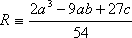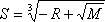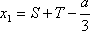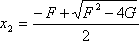# 11.5: Solution Techniques for Cubic Expressions and Root Finding

Hopefully, we have convinced you that the use of cubic equations of state can represent a very meaningful and advantageous way of modeling the PVT behavior of petroleum fluids. What we need now are the tools that will allow us to get the information that we want out of them. Even though cubic equations of state are explicit in pressure, pressure is not the common unknown to be calculated in the typical problem. In the most common problem, pressure and temperature are known and we want either molar volume (or its reciprocal, molar density) or compressibility factor (the most likely case). Therefore, we are faced very often with the need to solve for the roots of a cubic expression. Here we present a number of approaches that may be followed.

## Analytical scheme

Given the cubic polynomial with real coefficients: x3 + ax2 + bx + c = 0, the first step is to calculate the parameters:and(11.5)

Now let M = R2 – Q3 be the discriminant. We then consider the following cases:

1. If M < 0 (R2 < Q3), the polynomial has three real roots. For this case, computeand calculate the three distinct real roots as:(11.6a)(11.6b)(11.6c)

Note that x1, x2, x3 are not given in any special order, and thathas to be calculated in radians.

2. If M > 0 (R2 > Q3), the polynomial has only one real root. Compute:(11.7a)(11.7b)

and calculate the real root as follows:(11.7c)

Two complex roots (complex conjugates) may be found as well. However, they are of no interest for our purposes, and thus no formulas are provided. Such formulas can be found in the following suggested readings:

• W.H. Press, S.A. Teukolsky, W.T. Vetterling, B.P. Flannery, Numerical Recipes in Fortran, 2nd Edition, Cambridge Univ. Press, (1992), p. 179.
• Spiegel, M., Liu, J., Mathematical handbook of formulas and tables, 2nd Edition, Schaum’s Outline Series, McGraw Hill, p.10.

Sometimes, the equations for S and T listed above cause problems while programming. This usually happens whenever the computer/calculator performs the cubic root of a negative quantity. If you want to avoid such a situation, you may compute S’ and T’ instead:(making T’=0 when S’=0)

where:

abs(R) = Absolute value of R

and

sign(R) = (+1) or (– 1) if R is positive or negative respectively.

It may be defined as:

sign(R) = R/Abs(R)

and then the real root is:

Keep in mind the following useful relationships among the roots of any cubic expression:

x1 + x2 + x3 = – a(11.8a)

x1x2 + x2x3 + x3x1 = +b(11.8b)

x1 x2 x3 = – c.(11.8c)

## Self-Check

Test your understanding of cubic root calculations by analytical means by solving the following examples.

With general cubic expressions,

x3 – 6x2 + 11x – 6 = 0 =>
x1 = 1
x2 = 2
x3 = 3

x3 + 7x2 + 49x + 343 = 0
x1 = – 7
x2 = 0 + 7 i
x3 = 0 – 7 i

x3 + 2x2 + 3x + 4 = 0 =>
x1 = – 1 .65063
x2 = – 0.174685 + 1.54687 i
x3 = – 0.174685 – 1.54687 i

with EOS in terms of volume (v), for a pure component,

v3 – 7.8693 v2 + 13.3771 v – 6.5354 = 0

=> One real root (one phase),
v1 = 5.7357 v3 – 15.6368 v2 + 30.315v – 14.8104 = 0

=> Two possible phases (three real roots),
v1 = 0.807582 (liquid phase)
v2 = 1.36174 (rejected)
v3 = 13.4675(gas phase)

with EOS in terms of the compressibility factor (z), for a pure component,

## z3 – 1.0595 z2 + 0.2215 z – 0.01317 = 0 => One phase, z = 0.8045 z3 – z2 + 0.089 z – 0.0013 = 0 => Two possible phases, zliquid = 0.0183012 zx = 0.0786609 (useless) zvapor = 0.903038 Numerical Scheme

The Newton-Raphson method provides a useful scheme for solving for a non-explicit variable from any form of equation (not only cubic ones). Newton-Raphson is an iterative procedure with a fast convergence, although it is not always capable of providing an answer — because a first guess close enough to the actual answer must be provided.

In solving for “x” in any equation of the type f(x)=0, the method provides a new estimate (new guess) closer to the actual answer, based on the previous estimate (old guess). This is written as follows:(11.9)

Considering a cubic equation f(x) = x3 + ax2 + bx + c = 0, the previous equation takes the form:(11.10)

The iterations continue until no significant improvement for “xnew” is achieved, i.e., | xnew – xold | < tolerance. An educated guess must be provided as the starting value for the iterations. If you are solving a cubic equation in Z (compressibility factor), it is usually recommend to take Z = bP/RT as the starting guess for the compressibility of the liquid phase and Z = 1 for the vapor root.

## Semi-analytical Scheme

If you used the previous numerical approach to calculate one of the roots of the cubic expression, the semi-analytical scheme can give you the other two real roots (when they exist). By using the relationships given before, with the value ‘x1’ as the root already known, the other two roots are calculated by solving the system of equations:

x2 + x3 = – a – x1(11.11a)
x2x3 = – c/x1 (11.11b)

This procedure can be reduced to the following steps:

1. Let x3 + ax2 + bx + c = 0 be the original cubic polynomial and “E” the root which is already known (x1 = E). Then, we may factorize such a cubic expression as:

(x – E) (x2 + Fx + G) = 0(11.12a)
where:

F = a + E(11.12b)
G = – c / E(11.12c)

2. Solve for x2, x3 by using the quadratic expression formulae,

x1 = E (11.13a)(11.13b)(11.13b)

## Contributors

• Prof. Michael Adewumi (The Pennsylvania State University). Some or all of the content of this module was taken from Penn State's College of Earth and Mineral Sciences' OER Initiative.